JavaScript，通常缩写为 JS，是一种解释执行的编程语言。它是现在最流行的脚本语言之一。

JavaScript 是一门基于原型、函数先行的语言，是一门多范式的语言，它支持面向对象编程、命令式编程、函数式编程。

JavaScript 是属于 Web 的语言，它被设计为向 HTML 页面增加交互性。

## 快速入门

``````alert("Hello World!"); // 第一行 JavaScript 代码
``````

### 调试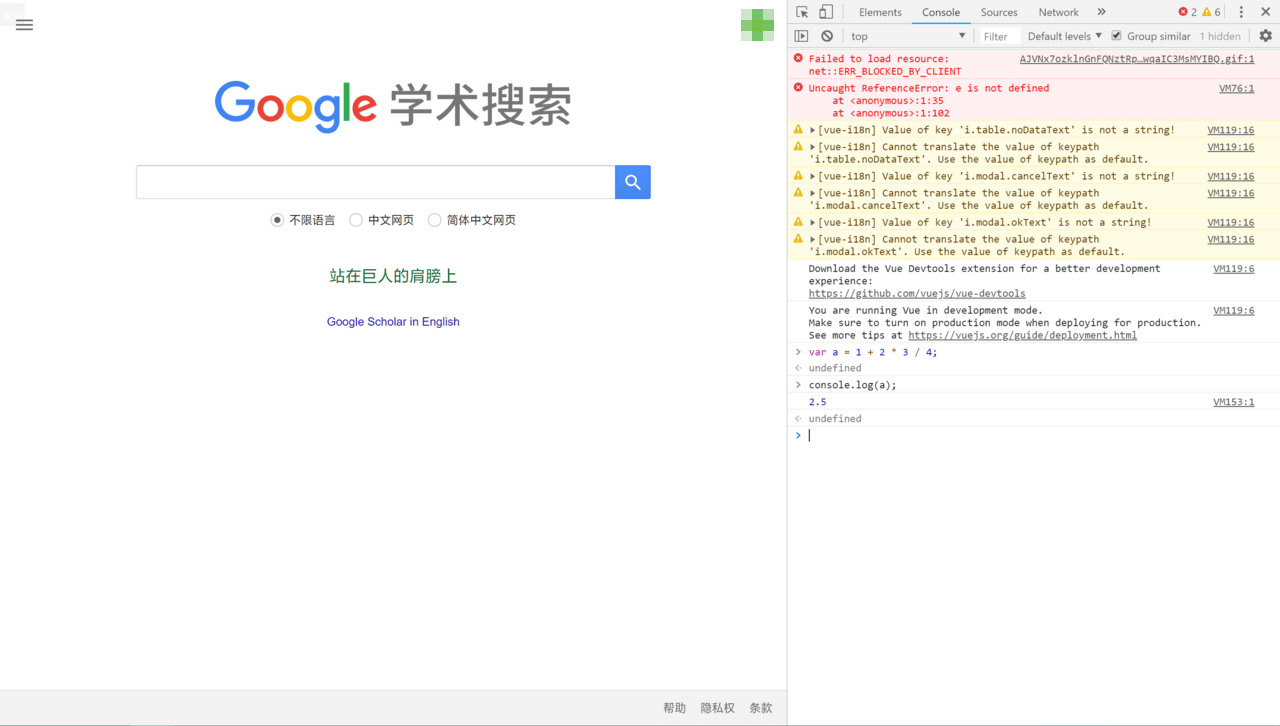### 数据类型和变量

#### 数字（number）

JavaScript 不区分整数和浮点数，统一用 number 表示，以下都是合法的 number 类型：

``````123;        // 整数 123
0.456;      // 浮点数 0.456
1.2345e3;   // 科学计数法表示 1.2345×1000，等同于 1234.5
-99;        // 负数
NaN;        // NaN 表示 Not a Number。当无法计算结果时用 NaN 表示
Infinity;   // Infinity 表示无穷大。当数值超过了 JavaScript 的 Number 所能表示的最大值时，就表示为 Infinity
``````

number 可以直接做四则运算，规则和数学一致：

``````(1 + 2) * 5 / 2;    // 7.5
2 / 0;              // Infinity
0 / 0;              // NaN
10 % 3;             // 1
``````

#### 比较运算符

``````false == 0;     // true
false === 0;    // false
``````

• 第一种是 `==` 比较，它会自动转换数据类型再比较。很多时候，会得到非常诡异的结果；

• 第二种是 `===` 比较，它不会自动转换数据类型。如果数据类型不一致，返回 `false`；如果一致，再比较。

``````NaN === NaN; // false
``````

``````isNaN(NaN); // true
``````

#### 空（null）和未定义（undefined）

`null` 表示一个“空”的值，`undefined` 表示值“未定义”。

#### 数组（array）

JavaScript 的数组可以包含任意数据类型。例如：

``````[1, 2, 3.14, "Hello", null, true];
``````

``````var arr = [1, 2, 3.14, "Hello", null, true];
arr; // 返回索引为 0 的元素，即 1
arr; // 返回索引为 5 的元素，即 true
arr; // 索引超出了范围，返回 undefined
``````

#### 对象（object）

JavaScript 的对象是一组由键－值组成的无序集合，例如：

``````var person = {
name: "Bob",
age: 20,
tags: ["js", "web", "mobile"],
city: "Beijing",
hasCar: true,
zipcode: null
};
``````

JavaScript 对象的键都是字符串类型，值可以是任意数据类型。上述 `person` 对象一共定义了 6 个键值对，其中每个键又称为对象的属性。例如，`person``name` 属性为 `"Bob"``zipcode` 属性为 `null`

#### 变量

``````var a = 123;    // a 的值是整数 123
a = "ABC";      // a 变为字符串 "ABC"
``````

``````int a = 123;  // a 是整数类型变量，类型用 int 声明
a = "ABC";    // 错误：不能把字符串赋给整型变量
``````

#### 严格模式

JavaScript 在设计之初，为了方便初学者学习，并不强制要求使用 `var` 声明变量。这个设计错误带来了严重的后果，未使用 `var` 声明的变量将会被视为全局变量：

``````i = 10; // i 现在是全局变量
``````

JavaScript 在后来推出了严格模式，强制要求使用 `var` 声明变量，否则将导致运行错误。

``````"use strict";
``````

### 字符串

#### 多行字符串

``````console.log(`多行

``````

#### 模板字符串

``````var name = "小明";
var age = 20;
var message = `你好，\${name}，你今年 \${age} 岁了！`;
console.log(message);
``````

#### 操作字符串

``````var s = "Test";
s = "X";
console.log(s); // s 仍然为 "Test"
``````

JavaScript 为字符串提供了一些常用方法。注意，调用这些方法本身不会改变原有字符串的内容，而是返回一个新字符串：

##### indexOf

`indexOf()` 会搜索指定字符串出现的位置：

``````var s = "hello, world";
s.indexOf("world"); // 返回 7，即子串的首字符的位置
s.indexOf("World"); // 没有找到指定的子串，返回 -1
``````
##### substring

`substring()` 返回指定索引区间的子串：

``````var s = "hello, world";
s.substring(0, 5);  // 从索引 0 开始到 5（不包括 5），返回 "hello"
s.substring(7);     // 从索引 7 开始到结束，返回 "world"
``````

### 数组

``````var arr = [1, 2, 3];
arr.length; // 3
arr.length = 6;
arr; // arr 加长为 [1, 2, 3, undefined, undefined, undefined]
arr.length = 2;
arr; // arr 缩短为 [1, 2]
``````

``````var arr = [1, 2, 3];
arr = "x";
arr; // arr 变为 [1, 2, 3, undefined, undefined, "x"]
``````

#### 数组的方法

##### slice

`slice()` 对应于对应字符串中的 `substring()`，它截取数组的部分元素，然后返回一个新的数组：

``````var arr = ["A", "B", "C", "D", "E", "F", "G"];
arr.slice(0, 3);    // 索引范围 [0, 3): ["A", "B", "C"]
arr.slice(3);       // 从索引 3 开始到结束: ["D", "E", "F", "G"]
``````

``````var arr = ["A", "B", "C", "D", "E", "F", "G"];
var aCopy = arr.slice();
aCopy;          // ["A", "B", "C", "D", "E", "F", "G"]
aCopy === arr;  // false
``````

``````var s = "Hello, World!";
var sCopy = s.substring();
sCopy; // "Hello, World!"
sCopy === s; // true
``````
##### push 和 pop

`push()` 向数组的末尾添加若干元素，`pop()` 则删除数组的末元素：

``````var arr = [1, 2];
arr.push("A", "B");                 // 返回数组新的长度: 4
arr;                                // [1, 2, "A", "B"]
arr.pop();                          // pop() 返回 "B"
arr;                                // [1, 2, "A"]
arr.pop(); arr.pop(); arr.pop();    // 连续 pop 三次
arr;                                // []
arr.pop();                          // 空数组继续 pop 不会报错，而是返回 undefined
arr;                                // []
``````
##### unshift 和 shift

`unshift()` 往数组的头部添加若干元素，`shift()` 则删除数组的首元素：

``````var arr = [1, 2];
arr.unshift("A", "B");                  // 返回数组新的长度: 4
arr;                                    // ["A", "B", 1, 2]
arr.shift();                            // "A"
arr;                                    // ["B", 1, 2]
arr.shift(); arr.shift(); arr.shift();  // 连续 shift 三次
arr;                                    // []
arr.shift();                            // 空数组继续 shift 不会报错，而是返回 undefined
arr;                                    // []
``````
##### sort

`sort()` 可以对当前数组进行排序，它会直接修改当前数组的元素位置。直接调用时，按照默认顺序排序：

``````var arr = ["B", "C", "A"];
arr.sort();
arr; // ["A", "B", "C"]
``````

##### reverse

`reverse()` 把整个数组的元素反转：

``````var arr = ["one", "two", "three"];
arr.reverse();
arr; // ["three", "two", "one"]
``````
##### splice

`splice()` 是修改数组的“万能方法”，它可以从指定的索引开始删除若干元素，然后再从该位置添加若干元素：

``````var arr = ["Microsoft", "Apple", "Yahoo", "AOL", "Excite", "Oracle"];
// 从索引 2 开始删除三个元素，然后再添加两个元素：
// 只删除，不添加：
arr;                                    // ["Microsoft", "Apple", "Oracle"]
// 只添加，不删除：
``````
##### concat

`concat()` 把当前数组和另一个数组连接起来，并返回一个新的数组：

``````var arr = ["A", "B", "C"];
var added = arr.concat([1, 2, 3]);
added;  // ["A", "B", "C", 1, 2, 3]
arr;    // ["A", "B", "C"]
``````

``````var arr = ["A", "B", "C"];
arr.concat(1, 2, [3, 4]); // ["A", "B", "C", 1, 2, 3, 4]
``````
##### join

`join()` 是一个非常实用的方法，它把当前数组的每个元素都用指定的字符串连接起来，然后返回连接后的字符串：

``````var arr = ["A", "B", "C", 1, 2, 3];
arr.join("-"); // "A-B-C-1-2-3"
``````

#### 多维数组

``````var arr = [[1, 2, 3], [400, 500, 600], "-"];
``````

### 对象

JavaScript 的对象是一种无序的集合数据类型，它由若干键值对组成。

``````var xiaohong = {
name: "小红",
"middle-school": "No.1 Middle School"
};
``````

`xiaohong` 的属性名 `middle-school` 不是一个有效的变量，就需要用引号括起来。访问这个属性也无法使用 `.` 操作符，必须用 `["xxx"]` 来访问：

``````xiaohong["middle-school"];  // "No.1 Middle School"
xiaohong["name"];           // "小红"
xiaohong.name;              // "小红"
``````

``````var xiaoming = {
name: "小明"
};
xiaoming.age;               // undefined
xiaoming.age = 18;          // 新增一个 age 属性
xiaoming.age;               // 18
delete xiaoming.age;        // 删除 age 属性
xiaoming.age;               // undefined
delete xiaoming["name"];    // 删除 name 属性
xiaoming.name;              // undefined
delete xiaoming.school;     // 删除一个不存在的 school 属性不会报错
``````

``````var xiaoming = {
name: "小明",
birth: 1990,
school: "No.1 Middle School",
height: 1.70,
weight: 65,
score: null
};
"name" in xiaoming;     // true
``````

``````"toString" in xiaoming; // true
``````

``````var xiaoming = {
name: "小明"
};
xiaoming.hasOwnProperty("name");        // true
xiaoming.hasOwnProperty("toString");    // false
``````

### 条件判断

`if (condition) { … }` 中，条件逻辑语句 `condition` 的结果有时不是布尔值。

JavaScript 把 `null``undefined``0``NaN` 和空字符串 `""` 视为 `false`，其它值一律视为 `true`

### 循环

#### for … in

`for` 循环的一个变体是 `for … in` 循环，它可以把一个对象的所有属性依次循环出来：

``````var o = {
name: "Jack",
age: 20,
city: "Beijing"
};
for (var key in o) {
console.log(key); // "name"，"age"，"city"
}
``````

``````var o = {
name: "Jack",
age: 20,
city: "Beijing"
};
for (var key in o) {
if (o.hasOwnProperty(key))
console.log(key); // "name"，"age"，"city"
}
``````

``````var a = ["A", "B", "C"];
for (var i in a) {
console.log(i);     // "0"，"1"，"2"
console.log(a[i]);  // "A"，"B"，"C"
}
``````

### Map 和 Set

JavaScript 默认的对象表示方式 `{}` 可以视为其它编程语言中的 `Map``Dictionary` 的数据结构，即一组键值对。

#### Map

`Map` 是一组键值对的结构，具有极快的查找速度。

``````var names = ["Michael", "Bob", "Tracy"];
var scores = [95, 75, 85];
``````

``````var m = new Map([
["Michael", 95],
["Bob", 75],
["Tracy", 85]
]);
m.get("Michael"); // 95
``````

``````var m = new Map();
``````

``````var m = new Map();
``````

#### Set

`Set``Map` 类似，也是一组键的集合，但不存储值。由于键不能重复，所以在 `Set` 中没有重复的键。

``````var s1 = new Set();             // 空 Set
var s2 = new Set([1, 2, 3]);    // 含 1、2、3
``````

``````var s = new Set([1, 2, 3, 3, "3"]);
s; // Set {1, 2, 3, "3"}
``````

``````s.add(4);
s; // Set {1, 2, 3, 4}
s; // 仍然是 Set {1, 2, 3, 4}
``````

``````var s = new Set([1, 2, 3]);
s; // Set {1, 2, 3}
s.delete(3);
s; // Set {1, 2}
``````

### 迭代（iterable）

`for … in` 循环由于历史遗留问题，它遍历的实际上是对象的属性名称。一个数组实际上也是一个对象，它的每个元素的索引被视为一个属性。

``````var a = ["A", "B", "C"];
a.name = "Hello";
for (var x in a) {
console.log(x); // "0", "1", "2", "name"
}
``````

`for … of` 循环则完全修复了这些问题，它只循环集合本身的元素：

``````var a = ["A", "B", "C"];
a.name = "Hello";
for (var x of a) {
console.log(x); // "A", "B", "C"
}
``````

``````var a = ["A", "B", "C"];
a.forEach(function (element, index, array) {
// element: 指向当前元素
// index: 指向当前索引
// array: 指向数组本身
console.log("[" + element + ", index = " + index + "]"); // [A, index = 0]，[B, index = 1]，[C, index = 2]
});
``````

`Set` 与数组类似，但 `Set` 没有索引，因此回调函数的前两个参数都是元素本身：

``````var s = new Set(["A", "B", "C"]);
s.forEach(function (element, sameElement, set) {
console.log(element); // "A"，"B"，"C"
});
``````

`Map` 的回调函数参数依次为值、键和 `Map` 本身：

``````var m = new Map([
[1, "x"],
[2, "y"],
[3, "z"]
]);
m.forEach(function (value, key, map) {
console.log(value); // "x"，"y"，"z"
});
``````

``````var a = ["A", "B", "C"];
a.forEach(function (element) {
console.log(element); // "A"，"B"，"C"
});
``````

``````var m = new Map([
["Michael", 95],
["Bob", 75],
["Tracy", 85]
]);
m.forEach(function (value, key) {
console.log(key); // "Michael"，"Bob"，"Tracy"
});
``````

## 函数

### 函数定义和调用

#### 定义函数

``````function abs(x) {
if (x >= 0) {
return x;
} else {
return -x;
}
}
``````

``````var abs = function (x) {
if (x >= 0) {
return x;
} else {
return -x;
}
};
``````

#### 调用函数

``````abs(); // 返回 NaN
``````

``````function abs(x) {
if (typeof x !== "number") {
throw "Not a Number";
}
if (x >= 0) {
return x;
} else {
return -x;
}
}
``````

#### arguments

JavaScript 有一个关键字 `arguments`，它只在函数内部起作用，并且指向当前函数的调用者传入的所有参数。`arguments` 类似数组但它不是一个数组：

``````function foo(x) {
console.log("x = " + x); // 10
for (var i = 0; i < arguments.length; i++) {
console.log("arg " + i + " = " + arguments[i]); // 10, 20, 30
}
}
foo(10, 20, 30);

/*
x = 10
arg 0 = 10
arg 1 = 20
arg 2 = 30
*/
``````

``````function abs() {
if (arguments.length === 0) {
return 0;
}
var x = arguments;
return x >= 0 ? x : -x;
}

abs();      // 0
abs(10);    // 10
abs(-9);    // 9
``````

``````// foo(a[, b], c)
// 接收 2～3 个参数，b 是可选参数。如果只传两个参数，b 默认为 null：
function foo(a, b, c) {
if (arguments.length === 2) {
// 实际拿到的参数是 a 和 b，c为 undefined
c = b; // 把 b 赋给c
b = null; // b 变为默认值
}
// …
}
``````

#### rest 参数

``````function foo(a, b) {
var i, rest = [];
if (arguments.length > 2) {
for (i = 2; i < arguments.length; i++) {
rest.push(arguments[i]);
}
}
console.log("a = " + a);
console.log("b = " + b);
console.log(rest);
}
``````

``````function foo(a, b, ...rest) {
console.log("a = " + a);
console.log("b = " + b);
console.log(rest);
}

foo(1, 2, 3, 4, 5);
/*
a = 1
b = 2
[ 3, 4, 5 ]
*/

foo(1);
/*
a = 1
b = undefined
[]
*/
``````

`rest` 参数只能写在最后，前面用 `...` 标识。从运行结果可知，传入的参数先绑定 `a``b`，多余的参数以数组形式交给变量 `rest`。所以不再需要 `arguments` 就获取了全部参数。

#### return 语句

``````function foo() {
return { name: "foo" };
}
foo(); // { name: "foo" }
``````

``````function foo() {
return
{ name: "foo" };
}
foo(); // undefined
``````

``````function foo() {
return; // 自动添加了分号，相当于 return undefined;
{ name: "foo" }; // 这行语句已经没法执行到了
}
``````

``````function foo() {
return { // 这里不会自动加分号，因为 { 表示语句尚未结束
name: "foo"
};
}
``````

### 变量作用域与解构赋值

#### 变量提升

JavaScript 在进行函数定义时，会把所有声明的变量“提升”到函数前部：

``````"use strict";

function foo() {
var x = "Hello, " + y;
console.log(x);
var y = "Bob";
}
foo();
``````

``````function foo() {
var y; // 提升变量 y 的声明，此时 y 为 undefined
var x = "Hello, " + y;
console.log(x);
y = "Bob";
}
``````

``````function foo() {
var
x = 1,      // x 初始化为 1
y = x + 1,  // y 初始化为 2
z, i;       // z 和 i 为 undefined
// 其他语句：
for (i = 0; i < 100; i++) {
// …
}
}
``````

#### 命名空间

``````// 唯一的全局变量 MYAPP：
var MYAPP = {};

// 其他变量：
MYAPP.name = "myapp";
MYAPP.version = 1.0;

// 其他函数：
MYAPP.foo = function () {
return "foo";
};
``````

#### 局部作用域

``````"use strict";

function foo() {
for (var i = 0; i < 100; i++) {
//
}
i += 100; // 仍然可以引用变量 i
}
``````

``````"use strict";

function foo() {
var sum = 0;
for (let i = 0; i < 100; i++) {
sum += i;
}
// SyntaxError:
i += 1;
}
``````

#### 解构赋值

``````var [x, y, z] = ["hello", "JavaScript", "ES6"];
// x、y、z 分别被赋值为数组对应元素：
console.log("x = " + x + ", y = " + y + ", z = " + z); // x = hello, y = JavaScript, z = ES6
``````

``````let [x, [y, [z]]] = ["hello", ["JavaScript", ["ES6"]]];
x; // "hello"
y; // "JavaScript"
z; // "ES6"
``````

``````let [, , z] = ["hello", "JavaScript", "ES6"]; // 忽略前两个元素，只对 z 赋值第三个元素
z; // "ES6"
``````

``````var person = {
name: "小明",
age: 20,
gender: "male",
passport: "G-12345678",
school: "No.4 middle school"
};
var {
name,
age,
passport
} = person;
// name、age、passport 分别被赋值为对应属性：
console.log("name = " + name + ", age = " + age + ", passport = " + passport); // name = 小明, age = 20, passport = "G-12345678"
``````

``````var person = {
name: "小明",
age: 20,
gender: "male",
passport: "G-12345678",
school: "No.4 middle school",
city: "Beijing",
zipcode: "100001"
}
};
var {
name,
city,
zip
}
} = person;
name; // "小明"
city; // "Beijing"
zip; // undefined。因为属性名是 zipcode 而不是 zip
``````

``````var person = {
name: "小明",
age: 20,
gender: "male",
passport: "G-12345678",
school: "No.4 middle school"
};

// 把 passport 属性赋值给变量 id：
let {
name,
passport: id
} = person;
name;   // "小明"
id;     // "G-12345678"
// 注意：passport 不是变量，而是为了让变量 id 获得 passport 属性：
passport; // Uncaught ReferenceError: passport is not defined
``````

``````var person = {
name: "小明",
age: 20,
gender: "male",
passport: "G-12345678"
};

// 如果 person 对象没有 single 属性，默认赋值为 true：
var {
name,
single = true
} = person;
name;   // "小明"
single; // true
``````

``````// 声明变量:
var x, y;
// 解构赋值:
{
x,
y
} = {
name: "小明",
x: 100,
y: 200
};
// 语法错误：Uncaught SyntaxError: Unexpected token =
``````

``````({
x,
y
} = {
name: "小明",
x: 100,
y: 200
});
``````

#### 使用场景

``````var x = 1,
y = 2;
[x, y] = [y, x];
``````

``````var {
hostname: domain,
pathname: path
} = location;
``````

``````function buildDate({
year,
month,
day,
hour = 0,
minute = 0,
second = 0
}) {
return new Date(year + "-" + month + "-" + day + " " + hour + ":" + minute + ":" + second);
}
``````

``````buildDate({
year: 2017,
month: 1,
day: 1
});
// Sun Jan 01 2017 00:00:00 GMT+0800 (中国标准时间)
``````

``````buildDate({
year: 2017,
month: 1,
day: 1,
hour: 20,
minute: 15
});
// Sun Jan 01 2017 20:15:00 GMT+0800 (中国标准时间)
``````

### 方法

``````"use strict";

var xiaoming = {
name: "小明",
birth: 1990,
age: function () {
var y = new Date().getFullYear();
return y - this.birth;
}
};

xiaoming.age(); // 28
var fn = xiaoming.age;
fn(); // Uncaught TypeError: Cannot read property "birth" of undefined
``````

``````"use strict";

var xiaoming = {
name: "小明",
birth: 1990,
age: function () {
function getAgeFromBirth() {
var y = new Date().getFullYear();
return y - this.birth;
}
return getAgeFromBirth();
}
};

xiaoming.age(); // Uncaught TypeError: Cannot read property "birth" of undefined
``````

``````"use strict";

var xiaoming = {
name: "小明",
birth: 1990,
age: function () {
var that = this;            // 在方法内部一开始就捕获 this
function getAgeFromBirth() {
var y = new Date().getFullYear();
return y - that.birth;  // 用 that 而不是 this
}
return getAgeFromBirth();
}
};

xiaoming.age(); // 25
``````

#### apply

`apply()` 修复 `getAge()` 调用：

``````function getAge() {
var y = new Date().getFullYear();
return y - this.birth;
}
var xiaoming = {
name: "小明",
birth: 1990,
age: getAge
};
xiaoming.age();             // 25
getAge.apply(xiaoming, []); // 25。this 指向 xiaoming，参数为空
``````

• `apply()` 把参数打包成数组再传入；

• `call()` 把参数按顺序传入。

``````Math.max.apply(null, [3, 5, 4]);    // 5
Math.max.call(null, 3, 5, 4);       // 5
``````

#### 装饰器

JavaScript 的所有对象都是动态的。即使是内置的函数，也可以重新指向新的函数。

``````var count = 0;
var oldParseInt = parseInt; // 保存原函数
window.parseInt = function () {
count += 1;
return oldParseInt.apply(null, arguments); // 调用原函数
};

// 测试：
parseInt("10");
parseInt("20");
parseInt("30");
console.log("count = " + count); // 3
``````

### 高阶函数

``````function add(x, y, f) {
return f(x) + f(y);
}
var x = add(-5, 6, Math.abs);
console.log(x); // 11
``````

#### map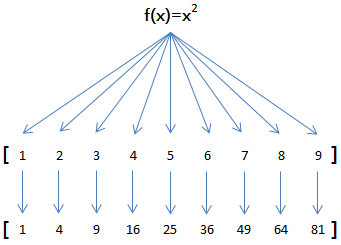``````function pow(x) {
return x * x;
}
var arr = [1, 2, 3, 4, 5, 6, 7, 8, 9];
var results = arr.map(pow);
console.log(results); // [1, 4, 9, 16, 25, 36, 49, 64, 81]
``````

#### reduce

``````[x1, x2, x3, x4].reduce(f) = f(f(f(x1, x2), x3), x4)
``````

``````function add(x, y) {
return x + y;
}
var arr = [1, 3, 5, 7, 9];
console.log(results); // 25
``````

#### 过滤（filter）

`filter` 也是一个常用的操作，它用于把数组的某些元素过滤掉，然后返回剩下的元素。

`map()` 类似，数组的 `filter()` 也接收一个函数。不同的是，`filter()` 把传入的函数依次作用于每个元素，然后根据返回值是 `true` 还是 `false` 决定保留还是丢弃该元素。

``````var arr = [1, 2, 4, 5, 6, 9, 10, 15];
var r = arr.filter(function (x) {
return x % 2 !== 0;
});
r; // [1, 5, 9, 15]
``````

``````var arr = ["A", "", "B", null, undefined, "C", "  "];
var r = arr.filter(function (s) {
return s && s.trim();
});
r; // ["A", "B", "C"]
``````

`filter()` 接收的回调函数，其实可以有多个参数。通常仅使用第一个参数，表示数组的某个元素。回调函数还可以接收另外两个参数，表示元素的位置和数组本身：

``````var arr = ["A", "B", "C"];
var r = arr.filter(function (element, index, self) {
console.log(element); // 依次打印 "A", "B", "C"
console.log(index); // 依次打印 0, 1, 2
console.log(self); // self 就是变量 arr
return true;
});
``````

``````var
r,
arr = ["apple", "strawberry", "banana", "pear", "apple", "orange", "orange", "strawberry"];
r = arr.filter(function (element, index, self) {
return self.indexOf(element) === index;
});
``````

#### 排序（sort）

JavaScript 数组的 `sort()` 方法就是用于排序的，但是排序结果可能出乎意料：

``````// 看上去正常的结果:

// apple 排在了最后:

// 无法理解的结果:
[10, 20, 1, 2].sort(); // [1, 10, 2, 20]
``````

``````var arr = [10, 20, 1, 2];
arr.sort(function (x, y) {
if (x < y) return -1;
if (x > y) return 1;
return 0;
});
console.log(arr); // [1, 2, 10, 20]
``````

### 闭包（closure）

#### 函数作为返回值

``````function sum(arr) {
return arr.reduce(function (x, y) {
return x + y;
});
}
sum([1, 2, 3, 4, 5]); // 15
``````

``````function lazy_sum(arr) {
var sum = function () {
return arr.reduce(function (x, y) {
return x + y;
});
}
return sum;
}
``````

``````var f = lazy_sum([1, 2, 3, 4, 5]); // 函数 sum()
``````

``````f(); // 15
``````

``````var f1 = lazy_sum([1, 2, 3, 4, 5]);
var f2 = lazy_sum([1, 2, 3, 4, 5]);
f1 === f2; // false
``````

#### 闭包

``````function count() {
var arr = [];
for (var i = 1; i <= 3; i++) {
arr.push(function () {
return i * i;
});
}
return arr;
}

var results = count();
var f1 = results;
var f2 = results;
var f3 = results;
``````

``````f1(); // 16
f2(); // 16
f3(); // 16
``````

``````function count() {
var arr = [];
for (var i = 1; i <= 3; i++) {
arr.push((function (n) {
return function () {
return n * n;
}
})(i));
}
return arr;
}

var results = count();
var f1 = results;
var f2 = results;
var f3 = results;

f1(); // 1
f2(); // 4
f3(); // 9
``````

``````(function (x) {
return x * x;
})(3); // 9
``````

``````"use strict";

function create_counter(initial) {
var x = initial || 0;
return {
inc: function () {
x += 1;
return x;
}
}
}
``````

``````var c1 = create_counter();
c1.inc(); // 1
c1.inc(); // 2
c1.inc(); // 3

var c2 = create_counter(10);
c2.inc(); // 11
c2.inc(); // 12
c2.inc(); // 13
``````

``````"use strict";

function make_pow(n) {
return function (x) {
return Math.pow(x, n);
}
}

// 创建两个新函数:
var pow2 = make_pow(2);
var pow3 = make_pow(3);

console.log(pow2(5)); // 25
console.log(pow3(7)); // 343
``````

### 箭头函数

JavaScript 中，函数可以用箭头语法（=>）定义，又称“Lambda 表达式”。即用类似于如下的表达式：

``````x => x * x;
``````

``````function (x) {
return x * x;
}
``````

``````x => {
if (x > 0) {
return x * x;
} else {
return -x * x;
}
}
``````

``````// 两个参数：
(x, y) => x * x + y * y;

// 无参数：
() => 3.14;

// 可变参数：
(x, y, ...rest) => {
var i, sum = x + y;
for (i = 0; i < rest.length; i++) {
sum += rest[i];
}
return sum;
}
``````

``````// SyntaxError:
x => {
foo: x
}
``````

``````x => ({
foo: x
})
``````

#### this

``````var obj = {
birth: 1990,
getAge: function () {
var b = this.birth; // 1990
var fn = function () {
return new Date().getFullYear() - this.birth; // this 指向 window 或 undefined
};
return fn();
}
};
``````

``````var obj = {
birth: 1990,
getAge: function () {
var b = this.birth; // 1990
var fn = () => new Date().getFullYear() - this.birth; // this 指向 obj 对象
return fn();
}
};
obj.getAge(); // 25
``````

``````var that = this;
``````

``````var obj = {
birth: 1990,
getAge: function (year) {
var b = this.birth; // 1990
var fn = (y) => y - this.birth; // this.birth 仍是 1990
return fn.call({ birth: 2000 }, year);
}
};
obj.getAge(2018); // 28
``````

### 生成器（generator）

``````function* foo(x) {
yield x + 1;
yield x + 2;
return x + 3;
}
``````

``````0, 1, 1, 2, 3, 5, 8, 13, 21, 34, ...
``````

``````function fib(max) {
var
t,
a = 0,
b = 1,
arr = [0, 1];
while (arr.length < max) {
[a, b] = [b, a + b];
arr.push(b);
}
return arr;
}

fib(5);     // [0, 1, 1, 2, 3]
fib(10);    // [0, 1, 1, 2, 3, 5, 8, 13, 21, 34]
``````

``````function* fib(max) {
var
t,
a = 0,
b = 1,
n = 0;
while (n < max) {
yield a;
[a, b] = [b, a + b];
n++;
}
return;
}
``````

``````fib(5); // fib {[[GeneratorStatus]]: "suspended", [[GeneratorReceiver]]: Window}
``````

``````var f = fib(5);
f.next(); // {value: 0, done: false}
f.next(); // {value: 1, done: false}
f.next(); // {value: 1, done: false}
f.next(); // {value: 2, done: false}
f.next(); // {value: 3, done: false}
f.next(); // {value: undefined, done: true}
``````

`next()` 方法会执行生成器的代码，然后每次遇到 `yield x;` 就返回一个对象 `{value: x, done: true/false}`，然后“暂停”。返回的 `value` 就是 `yield` 的返回值，`done` 表示这个生成器是否已经执行结束了。如果 `done``true`，则 `value` 就是 `return` 的返回值。

``````function* fib(max) {
var
t,
a = 0,
b = 1,
n = 0;
while (n < max) {
yield a;
[a, b] = [b, a + b];
n++;
}
return;
}
for (var x of fib(10)) {
console.log(x); // 依次输出 0, 1, 1, 2, 3, …
}
``````

``````var fib = {
a: 0,
b: 1,
n: 0,
max: 5,
next: function () {
var
r = this.a,
t = this.a + this.b;
this.a = this.b;
this.b = t;
if (this.n < this.max) {
this.n ++;
return r;
} else {
return undefined;
}
}
};
``````

``````ajax("http://url-1", data1, function (err, result) {
if (err) {
return handle(err);
}
ajax("http://url-2", data2, function (err, result) {
if (err) {
return handle(err);
}
ajax("http://url-3", data3, function (err, result) {
if (err) {
return handle(err);
}
return success(result);
});
});
});
``````

``````try {
r1 = yield ajax("http://url-1", data1);
r2 = yield ajax("http://url-2", data2);
r3 = yield ajax("http://url-3", data3);
success(r3);
}
catch (err) {
handle(err);
}
``````

## 标准对象

``````typeof 123; // "number"
typeof NaN; // "number"
typeof "str"; // "string"
typeof true; // "boolean"
typeof undefined; // "undefined"
typeof Math.abs; // "function"
typeof null; // "object"
typeof []; // "object"
typeof {}; // "object"
``````

### 包装对象

number、boolean 和 string 都有包装对象。包装对象用 `new` 创建：

``````var n = new Number(123);   // 123，生成了新的包装类型
var b = new Boolean(true); // true，生成了新的包装类型
var s = new String("str"); // "str"，生成了新的包装类型
``````

``````typeof new Number(123);  // "object"
new Number(123) === 123; // false

typeof new Boolean(true);   // "object"
new Boolean(true) === true; // false

typeof new String("str");    // "object"
new String("str") === "str"; // false
``````

``````var n = Number("123"); // 123，相当于 parseInt() 或 parseFloat()
typeof n; // "number"

var b = Boolean("true"); // true
typeof b; // "boolean"

var b2 = Boolean("false"); // true。"false" 字符串转换结果为 true，因为它是非空字符串
var b3 = Boolean(""); // false

var s = String(123.45); // "123.45"
typeof s; // "string"
``````

• 不要使用 `new Number()``new Boolean()``new String()` 创建包装对象；
• `parseInt()``parseFloat()` 来转换任意类型到 number；
• `String()` 来转换任意类型到 string，或者直接调用某个对象的 `toString()` 方法；
• 通常不必把任意类型转换为 boolean 再判断，因为可以直接写 `if (myVar) {…}`
• `typeof` 操作符可以判断出 number、boolean、string、function 和 undefined；
• 判断数组要使用 `Array.isArray(arr)`
• 判断 null 请使用 `myVar === null`
• 判断某个全局变量是否存在用 `typeof window.myVar === "undefined"`
• 函数内部判断某个变量是否存在用 `typeof myVar === "undefined"`

• null 和 undefined 没有 `toString()` 方法；

• number 对象调用 `toString()` 方法时需要以如下格式：

``````(123).toString(); // "123"
``````

#### Date

Date 对象表示日期和时间。

``````var now = new Date();
now; // Tue Jul 31 2018 20:08:50 GMT+0800 (中国标准时间)
now.getFullYear(); // 2018，年份
now.getMonth(); // 6，月份。注意月份范围是 0～11，6 表示七月
now.getDate(); // 31，表示 31 号
now.getDay(); // 2，表示星期二
now.getHours(); // 20，24 小时制
now.getMinutes(); // 8，分钟
now.getSeconds(); // 50，秒
now.getMilliseconds(); // 245，毫秒数
now.getTime(); // 1533038930245, 以 number 形式表示的时间戳
``````

``````var d = new Date(2018, 7, 29, 12, 30, 15, 123);
d; // Sun Jul 29 2018 12:30:15 GMT+0800 (中国标准时间)
``````

``````var d = new Date("2018-07-29T12:30:15.123+08:00");
d; // 1532838615123
``````

``````var d = new Date(1532838615123);
d; // Sun Jul 29 2018 12:30:15 GMT+0800 (中国标准时间)
``````

#### 时区

Date 对象表示的时间总是按浏览器所在时区显示的。不过既可以显示本地时间，也可以显示调整后的 UTC 时间：

``````var k = new Date(1532838615123);
k.toLocaleString(); // "2018/7/29 下午12:30:15"。本地时间（北京时区 +8:00），显示的字符串与操作系统设定的格式有关
k.toUTCString(); // "Sun, 29 Jul 2018 04:30:15 GMT"。UTC 时间，与本地时间相差 8 小时
``````

``````Date.now(); // 1533041129055
``````

### 正则表达式（RegExp）

JavaScript 有两种方式创建一个正则表达式：

``````var re1 = /ABC\-001/;
var re2 = new RegExp("ABC\\-001");

re1; // /ABC\-001/
re2; // /ABC\-001/
``````

``````var re = /^\d{3}\-\d{3,8}\$/;
re.test("010-12345"); // true
re.test("010-1234x"); // false
re.test("010 12345"); // false
``````

RegExp 对象的 `test()` 方法用于测试给定的字符串是否符合条件。

#### 切分字符串

``````"a b   c".split(" "); // ["a", "b", "", "", "c"]
``````

``````"a b   c".split(/\s+/); // ["a", "b", "c"]
``````

``````"a,b, c  d".split(/[\s\,]+/); // ["a", "b", "c", "d"]
``````

``````"a,b;; c  d".split(/[\s\,\;]+/); // ["a", "b", "c", "d"]
``````

#### 分组

`^(\d{3})-(\d{3,8})\$` 分别定义了两个组，可以直接从匹配的字符串中提取出区号和本地号码：

``````var re = /^(\d{3})-(\d{3,8})\$/;
re.exec("010-12345"); // ["010-12345", "010", "12345"]
re.exec("010 12345"); // null
``````

• `exec()` 方法在匹配成功后，会返回一个数组。第一个元素是正则表达式匹配到的整个字符串，后面的字符串表示匹配成功的子串。
• `exec()` 方法在匹配失败时返回 null。

``````var re = /^(0[0-9]|1[0-9]|2[0-3]|[0-9])\:(0[0-9]|1[0-9]|2[0-9]|3[0-9]|4[0-9]|5[0-9]|[0-9])\:(0[0-9]|1[0-9]|2[0-9]|3[0-9]|4[0-9]|5[0-9]|[0-9])\$/;
re.exec("19:05:30"); // ["19:05:30", "19", "05", "30"]
``````

``````var re = /^(0[1-9]|1[0-2]|[0-9])-(0[1-9]|1[0-9]|2[0-9]|3[0-1]|[0-9])\$/;
``````

#### 贪婪匹配

``````var re = /^(\d+)(0*)\$/;
re.exec("102300"); // ["102300", "102300", ""]
``````

``````var re = /^(\d+?)(0*)\$/;
re.exec("102300"); // ["102300", "1023", "00"]
``````

#### 全局搜索

JavaScript 的正则表达式还有几个特殊的标志，最常用的是 `g`，表示全局匹配：

``````var r1 = /test/g;
// 等价于：
var r2 = new RegExp("test", "g");
``````

``````var s = "JavaScript, VBScript, JScript and ECMAScript";
var re = /[a-zA-Z]+Script/g;

// 使用全局匹配：
re.exec(s); // ["JavaScript"]
re.lastIndex; // 10

re.exec(s); // ["VBScript"]
re.lastIndex; // 20

re.exec(s); // ["JScript"]
re.lastIndex; // 29

re.exec(s); // ["ECMAScript"]
re.lastIndex; // 44

re.exec(s); // null，直到结束仍没有匹配到
``````

## 面向对象编程

``````var Student = {
name: "Robot",
height: 1.2,
run: function () {
console.log(this.name + " is running…");
}
};
``````

``````var xiaoming = {
name: "小明"
};
Object.setPrototypeOf(xiaoming, Student);
``````

``````xiaoming.name;  // "小明"
xiaoming.run(); // 小明 is running…
``````

`xiaoming` 有自己的 `name` 属性，但并没有定义 `run()` 方法。不过，由于小明是从 `Student` 继承而来，只要 `Student``run()` 方法，`xiaoming` 也可以调用：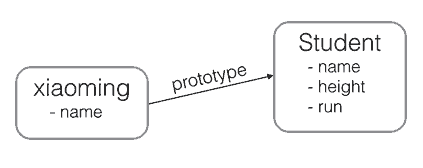JavaScript 的原型链和 Java、C# 中的类的区别就在于，它没有”类“的概念，所有对象都是实例，所谓继承关系不过是把一个对象的原型指向另一个对象而已。

``````var Bird = {
fly: function () {
console.log(this.name + " is flying…");
}
};
Object.setPrototypeOf(xiaoming, Bird);
``````

``````xiaoming.fly(); // 小明 is flying…
``````

`Object.create()` 方法可以传入一个原型对象，并创建一个基于该原型的新对象，但是新对象什么属性都没有，因此可以编写一个函数来创建 `xiaoming`

``````// 原型对象
var Student = {
name: "Robot",
height: 1.2,
run: function () {
console.log(this.name + " is running…");
}
};

function createStudent(iniName) {
var s = Object.create(Student); // 基于 Student 原型创建一个新对象
s.name = iniName; // 初始化新对象
return s;
}

var xiaoming = createStudent("小明");
xiaoming.run(); // 小明 is running…
Object.getPrototypeOf(xiaoming) === Student;
``````

### 创建对象

JavaScript 对每个创建的对象都会设置一个原型，指向它的原型对象。

``````var arr = [1, 2, 3];
``````

``````arr --> Array.prototype --> Object.prototype --> null
``````

`Array.prototype` 定义了 `indexOf()``shift()` 等方法，因此可以在所有的数组对象上直接调用这些方法。

``````function foo() {
return 0;
}
``````

``````foo --> Function.prototype --> Object.prototype --> null
``````

#### 构造函数

``````function Student(name) {
this.name = name;
this.hello = function () {
alert("Hello, " + this.name + "!");
};
}
``````

``````var xiaoming = new Student("小明");
xiaoming.name; // "小明"
xiaoming.hello(); // Hello, 小明!
``````

``````xiaoming --> Student.prototype --> Object.prototype --> null
``````

``````xiaoming ↘
xiaohong -→ Student.prototype --> Object.prototype --> null
xiaojun  ↗
``````

`new Student()` 创建的对象还从原型上获得了一个 `constructor` 属性，它指向函数 `Student` 本身：

``````xiaoming.constructor === Student.prototype.constructor; // true
Student.prototype.constructor === Student; // true
Object.getPrototypeOf(xiaoming) === Student.prototype; // true
xiaoming instanceof Student; // true
``````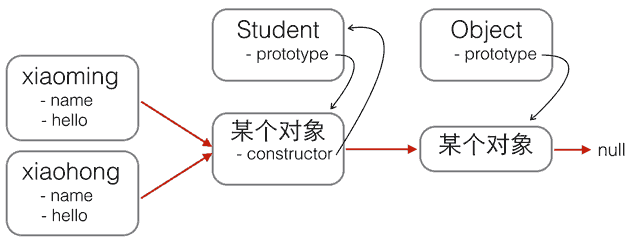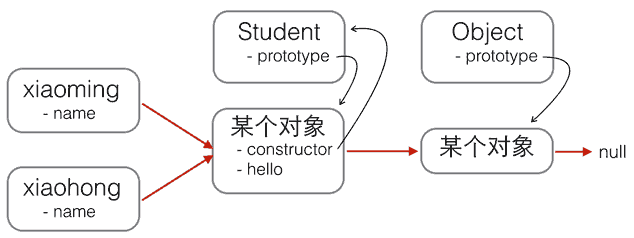``````function Student(name) {
this.name = name;
}
Student.prototype.hello = function () {
alert("Hello, " + this.name + "!");
};
``````

`new` 创建基于原型的 JavaScript 的对象就是这样。

#### 缺少 new 的构造函数

``````function Student(props) {
this.name = props.name || "匿名"; // 默认值为“匿名”
}

Student.prototype.hello = function () {
alert("Hello, " + this.name + "!");
};

function createStudent(props) {
return new Student(props || {});
}
``````

``````var xiaoming = createStudent({
name: "小明"
});

``````

### 原型继承

``````function Student(props) {
this.name = props.name || "Unnamed";
}

Student.prototype.hello = function () {
alert("Hello, " + this.name + "!");
};
``````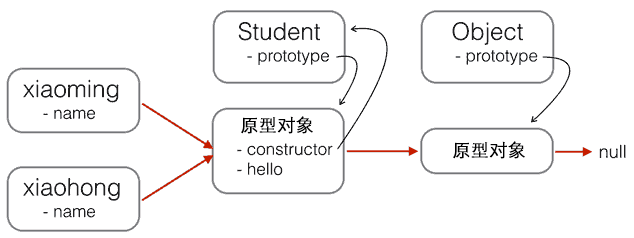``````function PrimaryStudent(props) {
// 调用 Student 构造函数，绑定 this 变量:
Student.call(this, props);
}
``````

``````new PrimaryStudent() --> PrimaryStudent.prototype --> Object.prototype --> null
``````

``````new PrimaryStudent() --> PrimaryStudent.prototype --> Student.prototype --> Object.prototype --> null
``````

``````PrimaryStudent.prototype = Object.create(Student.prototype);
PrimaryStudent.prototype.constructor = PrimaryStudent;
``````

``````// 创建 xiaoming
var xiaoming = new PrimaryStudent({
name: "小明",
});

xiaoming.name; // "小明"

// 验证原型：
Object.getPrototypeOf(xiaoming) === PrimaryStudent.prototype; // true
Object.getPrototypeOf(Object.getPrototypeOf(xiaoming)) === Student.prototype; // true

// 验证继承关系：
xiaoming instanceof PrimaryStudent; // true
xiaoming instanceof Student; // true
``````

### class 继承

class 的目的就是让定义类更简单。

``````function Student(name) {
this.name = name;
}

Student.prototype.hello = function () {
alert("Hello, " + this.name + "!");
};
``````

``````class Student {
constructor(name) {
this.name = name;
}
hello() {
alert("Hello, " + this.name + "!");
}
}
``````

``````var xiaoming = new Student("小明");
xiaoming.hello();
``````

``````class PrimaryStudent extends Student {
super(name); // 记得用 super() 调用基类的构造方法
}

}
}
``````

`PrimaryStudent` 自动获得了基类 `Student``hello()` 方法，其又定义了新的 `myGrade()` 方法。

## 浏览器

### 浏览器对象

JavaScript 可以获取浏览器提供的很多对象，并进行操作。

#### window

`window` 对象不但充当全局作用域，而且表示浏览器窗口。

`window` 对象有 `innerWidth``innerHeight` 属性，可以获取浏览器窗口的内部宽度和高度。内部宽高是指除去菜单栏、工具栏、边框等占位元素后，用于显示网页的净宽高，包括滚动条在内。

`navigator` 对象表示浏览器的信息，最常用的属性包括：

• `navigator.appName`：浏览器名称；
• `navigator.appVersion`：浏览器版本；
• `navigator.language`：浏览器设置的语言；
• `navigator.platform`：操作系统类型；
• `navigator.userAgent`：浏览器设定的用户代理字符串。

``````var width;
if (getIEVersion(navigator.userAgent) < 9) {
width = document.body.clientWidth;
} else {
width = window.innerWidth;
}
``````

``````var width = window.innerWidth || document.body.clientWidth;
``````

#### screen

`screen` 对象表示屏幕的信息，常用的属性有：

• `screen.width`：屏幕宽度，以像素为单位；
• `screen.height`：屏幕高度，以像素为单位；
• `screen.colorDepth`：颜色深度，如 8、16、24。根据 CSS 对象模型视图，为兼容起见，该值总为 24。

#### location

`location` 对象表示当前页面的 URL 信息。例如，一个完整的 URL：

``````http://www.example.com:8080/path/index.html?a=1&b=2#TOP
``````

``````location.protocol; // "http"
location.host; // "www.example.com"
location.port; // "8080"
location.pathname; // "/path/index.html"
location.search; // "?a=1&b=2"
location.hash; // "TOP"
``````

#### document

`document` 对象表示当前页面。由于 HTML 在浏览器中以 DOM 形式表示为树形结构，`document` 对象就是整个 DOM 树的根节点。

`document``title` 属性是从 HTML 文档中的 `<title>xxx</title>` 读取的，但是可以动态改变。

``````<dl id="drink-menu" style="border:solid 1px #ccc;padding:6px;">
<dt>摩卡</dt>
<dd>热摩卡咖啡</dd>
<dt>酸奶</dt>
<dd>北京老酸奶</dd>
<dt>果汁</dt>
<dd>鲜榨苹果汁</dd>
</dl>
``````

`document` 对象提供的 `getElementById()``getElementsByTagName()` 可以按 ID 获得一个 DOM 节点和按 Tag 名称获得一组 DOM 节点：

``````var menu = document.getElementById("drink-menu");
var drinks = document.getElementsByTagName("dt");

var s = "提供的饮料有：";
for (var i = 0; i < drinks.length; i++) {
s += drinks[i].innerHTML;
if (i >= drinks.length - 1) continue;
s += "、";
}
console.log(s);
``````

``````提供的饮料有：摩卡，酸奶，果汁
``````

`document` 对象还有一个 `cookie` 属性，可以获取当前页面的 Cookie。

### 操作 DOM

• 更新：更新该 DOM 节点的内容，相当于更新了该 DOM 节点表示的 HTML 的内容；
• 遍历：遍历该 DOM 节点下的子节点，以便进行进一步操作；
• 添加：在该 DOM 节点下新增一个子节点，相当于动态增加了一个 HTML 节点；
• 删除：将该节点从 HTML 中删除，相当于删掉了该 DOM 节点的内容以及它包含的所有子节点。

``````// 返回 ID 为“test”的节点：
var test = document.getElementById("test");

// 先定位 ID 为“test-table”的节点，再返回其内部所有 tr 节点：
var trs = document.getElementById("test-table").getElementsByTagName("tr");

// 先定位 ID 为“test-div”的节点，再返回其内部所有 class 包含 red 的节点：
var reds = document.getElementById("test-div").getElementsByClassName("red");

// 获取节点 test 下的所有直属子节点：
var cs = test.children;

// 获取节点 test 下第一个、最后一个子节点：
var first = test.firstElementChild;
var last = test.lastElementChild;
``````

``````// 通过 querySelector 获取 ID 为 q1 的节点：
var q1 = document.querySelector("#q1");

// 通过 querySelectorAll 获取 q1 节点内的符合条件的所有节点：
var ps = q1.querySelectorAll("div.highlighted > p");
``````

#### 更新 DOM

``````// 获取 <p id="p-id">…</p>
var p = document.getElementById("p-id");
// 设置文本为 abc：
p.innerHTML = "ABC"; // <p id="p-id">ABC</p>
// 设置 HTML：
p.innerHTML = "ABC <span style=\"color:red\">RED</span> XYZ";
// <p>…</p> 的内部结构已修改
``````

`innerHTML` 时要注意，是否需要写入 HTML。如果写入的字符串是通过网络拿到了，要注意对字符编码来避免 XSS 攻击。

``````// 获取 <p id="p-id">…</p>
var p = document.getElementById("p-id");
// 设置文本：
// HTML 被自动编码，无法设置一个 <script> 节点：
``````

``````// 获取 <p id="p-id">…</p>
var p = document.getElementById("p-id");
// 设置 CSS：
p.style.color = "#ff0000";
p.style.fontSize = "20px";
``````

#### 插入 DOM

``````<!-- HTML 结构 -->
<p id="js">JavaScript</p>
<div id="list">
<p id="java">Java</p>
<p id="python">Python</p>
<p id="scheme">Scheme</p>
</div>
``````

`<p id="js">JavaScript</p>` 添加到 `<div id="list">` 的最后一项：

``````var
js = document.getElementById("js"),
list = document.getElementById("list");
list.appendChild(js);
``````

``````<!-- HTML 结构 -->
<div id="list">
<p id="java">Java</p>
<p id="python">Python</p>
<p id="scheme">Scheme</p>
<p id="js">JavaScript</p>
</div>
``````

``````var
list = document.getElementById("list"),
``````

``````<!-- HTML 结构 -->
<div id="list">
<p id="java">Java</p>
<p id="python">Python</p>
<p id="scheme">Scheme</p>
</div>
``````

``````var d = document.createElement("style");
d.setAttribute("type", "text/css");
d.innerHTML = "p { color: red }";
``````

##### insertBefore

``````<!-- HTML 结构 -->
<div id="list">
<p id="java">Java</p>
<p id="python">Python</p>
<p id="scheme">Scheme</p>
</div>
``````

``````var
list = document.getElementById("list"),
ref = document.getElementById("python"),
``````

``````<!-- HTML 结构 -->
<div id="list">
<p id="java">Java</p>
<p id="python">Python</p>
<p id="scheme">Scheme</p>
</div>
``````

``````var
i, c,
list = document.getElementById("list");
for (i = 0; i < list.children.length; i++) {
c = list.children[i]; // 获得第 i 个子节点
}
``````

#### 删除 DOM

``````// 获得待删除节点：
var self = document.getElementById("to-be-removed");
// 获得父节点：
var parent = self.parentElement;
// 删除：
var removed = parent.removeChild(self);
removed === self; // true
``````

``````<div id="parent">
<p>First</p>
<p>Second</p>
</div>
``````

``````var parent = document.getElementById("parent");
parent.removeChild(parent.children);
parent.removeChild(parent.children); // ← 浏览器报错
``````

### 操作表单

HTML 表单的输入控件主要有以下几种：

• 文本框，对应的 `<input type="text">`，用于输入文本；
• 口令框，对应的 `<input type="password">`，用于输入口令；
• 单选框，对应的 `<input type="radio">`，用于选择一项；
• 复选框，对应的 `<input type="checkbox">`，用于选择多项；
• 下拉框，对应的 `<select>`，用于选择一项；
• 隐藏文本，对应的 `<input type="hidden">`，用户不可见，但表单提交时会把隐藏文本发送到服务器。

#### 获取值

``````// <input type="text" id="email">
var input = document.getElementById("email");
input.value; // "用户输入的值"
``````

``````// <label><input type="radio" name="weekday" id="monday" value="1">Monday</label>
// <label><input type="radio" name="weekday" id="tuesday" value="2">Tuesday</label>
var mon = document.getElementById("monday");
var tue = document.getElementById("tuesday");
mon.value; // "1"
tue.value; // "2"
mon.checked; // true 或者 false
tue.checked; // true 或者 false
``````

#### 设置值

``````var input = document.getElementById("email");
input.value = "test@example.com"; // 文本框的内容已更新
``````

#### 提交表单

``````<!-- HTML -->
<form id="test-form">
<input type="text" name="test">
<button type="button" onclick="doSubmitForm()">Submit</button>
</form>

<script>
function doSubmitForm() {
var form = document.getElementById("test-form");
// 可以在此修改 form 的 input…
// 提交 form：
form.submit();
}
</script>
``````

``````<!-- HTML -->
<form id="test-form" onsubmit="return checkForm()">
<input type="text" name="test">
<button type="submit">Submit</button>
</form>

<script>
function checkForm() {
var form = document.getElementById("test-form");
// 可以在此修改 form 的 input…
// 继续下一步：
return true;
}
</script>
``````

``````<!-- HTML -->
<button type="submit">Submit</button>
</form>

<script>
function checkForm() {
// 把用户输入的明文变为 MD5：
pwd.value = toMD5(pwd.value);
// 继续下一步：
return true;
}
</script>
``````

``````<!-- HTML -->
<button type="submit">Submit</button>
</form>

<script>
function checkForm() {
// 把用户输入的明文变为 MD5：
md5_pwd.value = toMD5(input_pwd.value);
// 继续下一步：
return true;
}
</script>
``````

### 操作文件

``````var f = document.getElementById("test-file-upload");
var filename = f.value;
if (!filename || !(filename.endsWith(".jpg") || filename.endsWith(".png") || filename.endsWith(".gif"))) {
return false;
}
``````

#### File API

HTML5 的 File API 提供了 `File``FileReader` 两个主要对象，可以获得文件信息并读取文件。

``````var
fileInput = document.getElementById("test-image-file"),
info = document.getElementById("test-file-info"),
preview = document.getElementById("test-image-preview");

// 监听 change 事件：
// 清除背景图片：
preview.style.backgroundImage = "";
// 检查文件是否选择：
if (!fileInput.value) {
info.innerHTML = "没有选择文件";
return;
}
// 获取 File 引用：
var file = fileInput.files;
// 获取 File 信息：
info.innerHTML = "文件：" + file.name + "<br>" +
"大小：" + file.size + "<br>" +
"修改：" + file.lastModifiedDate;
if (file.type !== "image/jpeg" && file.type !== "image/png" && file.type !== "image/gif") {
return;
}
// 读取文件：
var
data = e.target.result; // "data:image/jpeg;base64,/9j/4AAQSk…(base64编码)…"
preview.style.backgroundImage = "url(" + data + ")";
};
// 以 DataURL 的形式读取文件：
});
``````

#### 回调

``````reader.readAsDataURL(file);
``````

``````reader.onload = function(e) {
// 当文件读取完成后，自动调用此函数
};
``````

### AJAX

AJAX 全称 Asynchronous JavaScript and XML，意思是用 JavaScript 执行异步网络请求。

``````function success(text) {
var textarea = document.getElementById("test-response-text");
textarea.value = text;
}

function fail(code) {
var textarea = document.getElementById("test-response-text");
textarea.value = "Error code: " + code;
}

var request = new XMLHttpRequest(); // 新建 XMLHttpRequest 对象

request.onreadystatechange = function () { // 状态发生变化时，函数被回调
if (request.readyState === 4) { // 成功完成
// 判断响应结果：
if (request.status === 200) {
// 成功，通过 responseText 拿到响应的文本：
return success(request.responseText);
} else {
// 失败，根据响应码判断失败原因：
return fail(request.status);
}
} else {
// HTTP 请求还在继续……
}
};

// 发送请求:
request.open("GET", "/api/categories");
request.send();

``````

`XMLHttpRequest` 对象的 `open()` 方法有 3 个参数：第一个参数指定是 `GET` 还是 `POST`；第二个参数指定 URL 地址；第三个参数指定是否使用异步，默认是 true，所以不用写。

#### 安全限制

``````"/proxy?url=http://www.sina.com.cn"
``````

``````<html>
<script src="http://example.com/abc.js"></script>
…
<body>
…
</body>
</html>
``````

JSONP 通常以函数调用的形式返回。例如，返回 JavaScript 内容如下：

``````foo("data");
``````

``````refreshPrice({"0000001":{"code": "0000001", … });
``````

``````function refreshPrice(data) {
var p = document.getElementById("test-jsonp");
p.innerHTML = "当前价格：" +
data["0000001"].name + "：" +
data["0000001"].price + "；" +
data["1399001"].name + "：" +
data["1399001"].price;
}
``````

``````function getPrice() {
var
js = document.createElement("script"),
js.src = "https://api.money.126.net/data/feed/0000001,1399001?callback=refreshPrice";
}
``````

#### CORS

CORS 全称 Cross-Origin Resource Sharing，是 HTML5 规范定义的如何跨域访问资源。

Origin 表示本域，也就是浏览器当前页面的域。当 JavaScript 向外域（如 sina.com）发起请求后，浏览器收到响应后，首先检查 `Access-Control-Allow-Origin` 是否包含本域。如果是，则此次跨域请求成功；如果不是，则请求失败，JavaScript 将无法获取到响应的任何数据。用图来表示就是：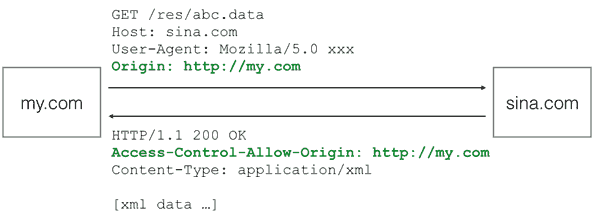``````/* CSS */

@font-face {
font-family: "FontAwesome";
src: url("http://cdn.com/fonts/fontawesome.ttf") format("truetype");
}
``````

``````OPTIONS /path/to/resource HTTP/1.1
Host: bar.com
Origin: http://my.com
Access-Control-Request-Method: POST
``````

``````HTTP/1.1 200 OK
Access-Control-Allow-Origin: http://my.com
Access-Control-Allow-Methods: POST, GET, PUT, OPTIONS
Access-Control-Max-Age: 86400
``````

### Promise

``````function callback() {
console.log("Done");
}
console.log("before setTimeout()");
setTimeout(callback, 1000); // 1 秒后调用 callback() 函数
console.log("after setTimeout()");
``````

``````before setTimeout()
after setTimeout()
// 等待 1 秒后
Done
``````

AJAX 就是典型的异步操作。以上一节的代码为例：

``````request.onreadystatechange = function () {
if (request.status === 200) {
return success(request.responseText);
} else {
return fail(request.status);
}
}
};
``````

``````var ajax = ajaxGet("http://…");
ajax.ifSuccess(success)
.ifFail(fail);
``````

``````function test(resolve, reject) {
var timeOut = Math.random() * 2;
log("set timeout to: " + timeOut + " seconds.");
setTimeout(function () {
if (timeOut < 1) {
log("call resolve()…");
resolve("200 OK");
} else {
log("call reject()…");
reject("timeout in " + timeOut + " seconds.");
}
}, timeOut * 1000);
}
``````

``````var p1 = new Promise(test);
var p2 = p1.then(function (result) {
console.log("成功：" + result);
});
var p3 = p2.catch(function (reason) {
console.log("失败：" + reason);
});
``````

``````// 如果成功，执行这个函数：
p1.then(function (result) {
console.log("成功：" + result);
});
``````

`test()` 函数执行失败时，告诉 Promise 对象：

``````p2.catch(function (reason) {
console.log("失败：" + reason);
});
``````

Promise 对象可以串联起来，所以上述代码可以简化为：

``````new Promise(test).then(function (result) {
console.log("成功：" + result);
}).catch(function (reason) {
console.log("失败：" + reason);
});
``````

``````// 清除 log：
var logging = document.getElementById("test-promise-log");
while (logging.children.length > 1) {
logging.removeChild(logging.children[logging.children.length - 1]);
}

// 输出 log 到页面：
function log(s) {
var p = document.createElement("p");
p.innerHTML = s;
logging.appendChild(p);
}

new Promise(function (resolve, reject) {
log("start new Promise…");
var timeOut = Math.random() * 2;
log("set timeout to: " + timeOut + " seconds.");
setTimeout(function () {
if (timeOut < 1) {
log("call resolve()…");
resolve("200 OK");
} else {
log("call reject()…");
reject("timeout in " + timeOut + " seconds.");
}
}, timeOut * 1000);
}).then(function (r) {
log("Done: " + r);
}).catch(function (reason) {
log("Failed: " + reason);
});
``````

``````start new Promise…
set timeout to: 0.6643159176821918 seconds.
call resolve()…
Done: 200 OK
``````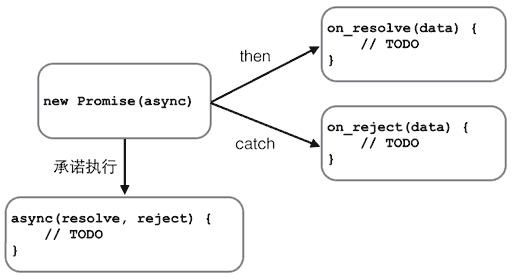Promise 还可以做更多的事情。比如，有若干个异步任务，需要先做任务 1，如果成功后再做任务 2，任何任务失败则不再继续并执行错误处理函数。

``````job1.then(job2).then(job3).catch(handleError);
``````

``````var logging = document.getElementById("test-promise2-log");
while (logging.children.length > 1) {
logging.removeChild(logging.children[logging.children.length - 1]);
}

function log(s) {
var p = document.createElement("p");
p.innerHTML = s;
logging.appendChild(p);
}

// 0.5 秒后返回 input × input 的计算结果:
function multiply(input) {
return new Promise(function (resolve, reject) {
log("calculating " + input + " x " + input + "…");
setTimeout(resolve, 500, input * input);
});
}

// 0.5 秒后返回 input + input 的计算结果:
return new Promise(function (resolve, reject) {
log("calculating " + input + " + " + input + "…");
setTimeout(resolve, 500, input + input);
});
}

var p = new Promise(function (resolve, reject) {
log("start new Promise…");
resolve(123);
});

p.then(multiply)
.then(multiply)
.then(function (result) {
log("Got value: " + result);
});
``````

``````start new Promise…
calculating 123 x 123…
calculating 15129 + 15129…
calculating 30258 x 30258…
calculating 915546564 + 915546564…
Got value: 1831093128
``````

`setTimeout()` 可以看成一个模拟网络等异步执行的函数。现在，把上一节的 AJAX 异步执行函数转换为 Promise 对象，看看用 Promise 如何简化异步处理：

``````// ajax() 函数将返回 Promise 对象
function ajax(method, url, data) {
var request = new XMLHttpRequest();
return new Promise(function (resolve, reject) {
if (request.status === 200) {
resolve(request.responseText);
} else {
reject(request.status);
}
}
};
request.open(method, url);
request.send(data);
});
}

var log = document.getElementById("test-promise-ajax-result");
var p = ajax("GET", "/api/categories");
p.then(function (text) { // 如果 AJAX 成功，获得响应内容
log.innerText = text;
}).catch(function (status) { // 如果 AJAX 失败，获得响应代码
log.innerText = "ERROR: " + status;
});
``````

``````{"categories":[{"id":"0013738748415562fee26e070fa4664ad926c8e30146c67000","name":"编程","tag":"tech","display_order":0,"description":"","created_at":1373874841556,"updated_at":1429763779958,"version":5},{"id":"0013738748248885ddf38d8cd1b4803aa74bcda32f853fd000","name":"读书","tag":"other","display_order":1,"description":"","created_at":1373874824888,"updated_at":1429763779974,"version":5}]}
``````

``````var p1 = new Promise(function (resolve, reject) {
setTimeout(resolve, 500, "P1");
});
var p2 = new Promise(function (resolve, reject) {
setTimeout(resolve, ``````